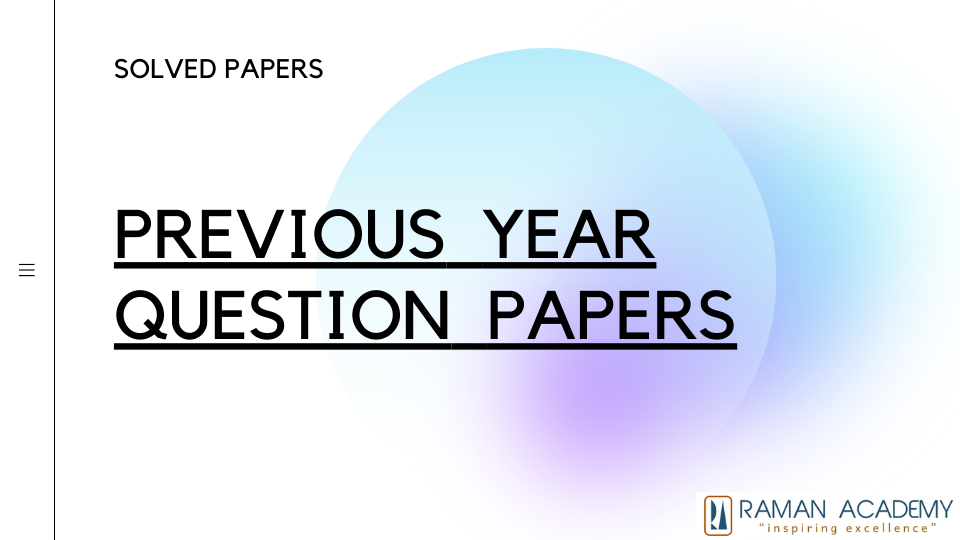# UPSC question paper – 2016-2020 – Mental Ability Analytical Logical Reasoning## Results

A)  Single dot

(b) Two dots

(c) Line

(d) Four dots

Ans C) Line

### #51. Rotated positions of a single solid are shown below. The various faces of the solid are marked with different symbols like dots, cross and line. Answer the three items that follow the given figures. What is the symbol on the face opposite to that containing two dots? 

(a) Single dot

(b) Three dots

(c) Four dots

(d) Line

Ans (c) Four dots

### #52. Rotated positions of a single solid are shown below. The various faces of the solid are marked with different symbols like dots, cross and line. Answer the three items that follow the given figures. What is the symbol on the face opposite to that containing a single dot? 

(a) Four dots

(b) Three dots

(c) Two dots

(d) Cross

Ans (b) Three dots

### #111. A person climbs a hill in a straight path from point ‘O’ on the ground in the direction of north-east and reaches a point ‘A’ after travelling a distance of 5 km. Then, from the point ‘A’ he moves to point ‘B’ in the direction of north- west. Let the distance AB be 12 km. Now, how far is the person away from the starting point ‘O’? 

Issues in Questions?

Tell us.!!